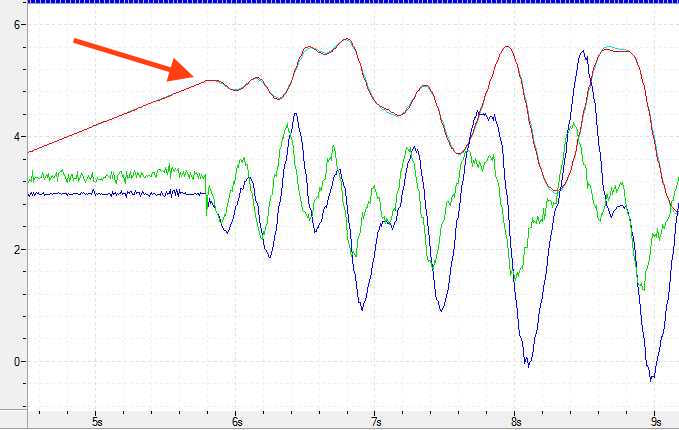# Ramping up a Track Position (57) command (SOLVED)

Using a RMC75E, I am starting a position sequence with the Move Absolute (20) to center stroke (pu 5.0). After waiting for “in Position”, the Track Position (57) is started. An Expression (113) will be calculating a summation of predetermined sine waves which are simulating the surface of the ocean, in a continuous motion, without discontinuities. However, there is a “discontinuity” , only at the moment the Expression (113) starts, after reaching center stroke. This “jerk” needs not to happen.

Yes… there are “limits” that are imposed to the Track Position (57), and the problem might be mitigated by experimenting with those limits (Velocity,Acceleration & jerk)

I’m wondering if this could be approached from a different direction … Is there a way to “Ramp Up” Expression (113)'s complex calculated wave form from zero; perhaps, as is done when ramping up the amplitude, or frequency of a simple sine wave? Regards, Michael

PS: The following was suggested by Jacob Pasco by email: “Yes, it is possible to ramp the signal at the start. I would specify a ramp time, say 1 second, and an offset, which you say is 5, and a percentage variable. I would make the final signal equal (percentage x summation) + ((100-percentage)*offset). The percentage would ramp from 0 to 100 over the ramp time. Therefore, at the beginning of the ramp, the final signal would be the offset (5), and at the end of the ramp time, the final signal would be just the summation.”

Yes, you can ramp from 5 to where ever your complicated formula says you should be but the track command should limit any jump in position.
If you want to ramp in the user program I use what I call a blended move. You have two targets. The first is the 5.0. The result of your formula. A first order blending would look like this
(1-A)*5+A)*formula_position=ramp_position
A starts at 0 and increments to 1 for a ramp time you specify.
This will probably work but there are higher order “blending” formulas that ramp from 0 to 1.

Hi Peter

Yes…and No… there is a price for avoiding that discontinuity at the start, after reaching pu 5.0. The resulting curve will be modified continuously thereafter, thus shifting much of the higher frequencies out of the spectrum.

Below is a curve with “jerk limit” at 10,000.
Running the same curve, with a “jerk limit” of 1000, the problem is gone; however the curve has changed. (it has also gotten larger… which is interesting. But, that’s for another time)

Jacob has suggested I plot the Master Signal to evaluate what’s going on … to be continued Regards, MichaelPeter
I think what you are suggesting, is the same as what Jacob has suggested ?..Sort of… Your formula is shown as: (1-A)*5+A)*formula_position=ramp_position
I’m assuming all those parentheses need to be there… but, we need one more… is it: (1-A)*5+(A)*formula_position=ramp_position ?
With A=zero yielding 5, and A=1 yields full formula_position. While 5 diminishes to zero on the way.

Now to create the work in RMC Tools… again, this is more fun than anyone should be allowed to have.